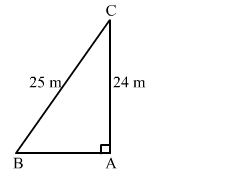# A ladder 25 m long just reaches the top of a building 24 m high from the ground.

Question:

A ladder 25 m long just reaches the top of a building 24 m high from the ground. What is the distance of the foot of the ladder from the building?
(a) 7 m
(b) 14 m
(c) 21 m
(d) 24.5 m

Solution:

(a) 7 mLet the ladder BC reaches the building at C.
Let the height of building where the ladder reaches be AC.
According to the question:
BC = 25 m
AC = 24 m
In right-angled triangle CAB, we apply Pythagoras theorem to find the value of AB.

$B C^{2}=A C^{2}+A B^{2}$

$\Rightarrow A B^{2}=B C^{2}-A C^{2}=25^{2}-24^{2}$

$\Rightarrow A B^{2}=625-576=49$

$\Rightarrow A B=\sqrt{49}=7 \mathrm{~m}$